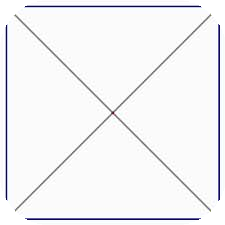### Python编程语言

Python 是一种面向对象、解释型计算机程序设计语言，由Guido van Rossum于1989年底发明，第一个公开发行版发行于1991年。Python语法简洁而清晰，具有丰富和强大的类库。它常被昵称为胶水语言，它能够把用其他语言制作的各种模块（尤其是C/C++）很轻松地联结在一起。import os, math
import Image
import ImageDraw

1 头像图片剪成圆形的，其他为透明

def circle():

ima = Image.open("test.jpg").convert("RGBA")

size = ima.size

# 因为是要圆形，所以需要正方形的图片

r2 = min(size, size)

if size != size:

ima = ima.resize((r2, r2), Image.ANTIALIAS)

imb = Image.new('RGBA', (r2, r2),(255,255,255,0))

r = float(r2/2) #圆心横坐标

for i in range(r2):

for j in range(r2):

lx = abs(i-r+0.5) #到圆心距离的横坐标

ly = abs(j-r+0.5)#到圆心距离的纵坐标

l  = pow(lx,2) + pow(ly,2)

if l <= pow(r, 2):

pimb[i,j] = pima[i,j]

imb.save("test_circle.png")

2、给图片的4个角加椭圆

def circle_corder_image():

im = Image.open("test.jpg").convert("RGBA")

draw = ImageDraw.Draw(circle)

alpha = Image.new('L', im.size, 255)

w, h = im.size

im.putalpha(alpha)

im.save('test_circle_corder.png')

def circle_new():

ima = Image.open("test.jpg").convert("RGBA")

size = ima.size

r2 = min(size, size)

if size != size:

ima = ima.resize((r2, r2), Image.ANTIALIAS)

circle = Image.new('L', (r2, r2), 0)

draw = ImageDraw.Draw(circle)

draw.ellipse((0, 0, r2, r2), fill=255)

alpha = Image.new('L', (r2, r2), 255)

alpha.paste(circle, (0, 0))

ima.putalpha(alpha)

ima.save('test_circle.png')

python处理圆角图片、圆形图片的例子
python实现DNS正向查询、反向查询的例子
Python处理json字符串转化为字典的简单实现
Python处理文本文件中控制字符的方法
python中使用pyhook实现键盘监控的例子
Python使用htpasswd实现基本认证授权的例子

python使用PyFetion来发送短信的例子

python生成圆形图片的方法
python实现下载给定网页上图片的方法
Python实现拼接多张图片的方法